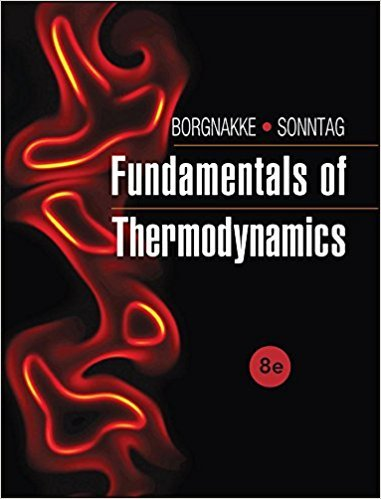×
Get Full Access to Fundamentals Of Thermodynamcs - 8 Edition - Chapter 3 - Problem 184hp
Get Full Access to Fundamentals Of Thermodynamcs - 8 Edition - Chapter 3 - Problem 184hp

×

# Air in a rigid tank is at 100 kPa, 300 K with a volume ofISBN: 9781118131992 58

## Solution for problem 184HP Chapter 3

Fundamentals of Thermodynamcs | 8th Edition

• Textbook Solutions
• 2901 Step-by-step solutions solved by professors and subject experts
• Get 24/7 help from StudySoup virtual teaching assistantsFundamentals of Thermodynamcs | 8th Edition

4 5 1 297 Reviews
14
1
Problem 184HP

Problem 184HP

Air in a rigid tank is at 100 kPa, 300 K with a volume of 0.75m3. The tank is heated to 400 K, state 2. Now one side of the tank acts as a piston, letting the air expand slowly at constant temperature to state 3 with a volume of 1.5 m3. Find the pressure at states 2 and 3. Find the total work and total heat transfer.

Step-by-Step Solution:

Solution 184HP

Step 1 of 6:

Our aim here is to find the pressure at the second and third states, total work and total heat transfer of the air filled tank.

The initial pressure P1 = 100 kPa

The initial temperature T1 = 300 K

The initial volume V1 = 0.75 m3

The gas constant R = 0.287 kJ/kg.K        (Table A.5)

The specific heat Cv0 = 0.717 kJ/kg.K        (Table A.5)

Step 2 of 6

Step 3 of 6

##### ISBN: 9781118131992

Fundamentals of Thermodynamcs was written by and is associated to the ISBN: 9781118131992. This textbook survival guide was created for the textbook: Fundamentals of Thermodynamcs , edition: 8. The answer to “Air in a rigid tank is at 100 kPa, 300 K with a volume of 0.75m3. The tank is heated to 400 K, state 2. Now one side of the tank acts as a piston, letting the air expand slowly at constant temperature to state 3 with a volume of 1.5 m3. Find the pressure at states 2 and 3. Find the total work and total heat transfer.” is broken down into a number of easy to follow steps, and 68 words. The full step-by-step solution to problem: 184HP from chapter: 3 was answered by , our top Engineering and Tech solution expert on 08/03/17, 05:05AM. This full solution covers the following key subjects: tank, air, Volume, state, Find. This expansive textbook survival guide covers 7 chapters, and 1462 solutions. Since the solution to 184HP from 3 chapter was answered, more than 1498 students have viewed the full step-by-step answer.

Unlock Textbook Solution